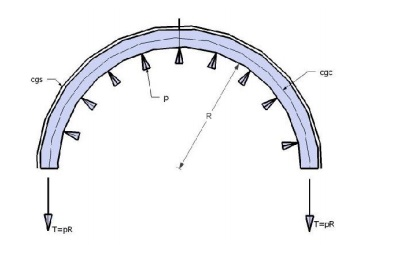Home | | Prestressed Concrete Structures | Circular pre stressing

# Circular pre stressingCircumferential pre-stressing is done to resist hoop tension in circular structures, like water-tanks and pipes.

Circular pre-stressing

Circumferential pre-stress

Circumferential pre-stressing is done to resist hoop tension in circular structures, like water-tanks and pipes. Essentially each horizontal slice of the wall forma a ring subjected to uniform internal pressure. This ring may be considered as a pre-stresses concrete member under tension.

Considering one half of a thin cylindrical slice of a tank as a free-body: under the action of pre-stress Fi in steel, the total compression C in the concrete equals Fi. The C-line coincides with the cgs line, which is a concordant cable linearly transformed.When internal liquid pressure is acting at working load stage, the internal pressure intensity,where

p = internal pressure intensity

R = internal radius of the vessel

AT = transformed area = Ac + nAp

AP = area of steel

The resultant stress in concrete at working load due to internal pressure 'p' and pre-stress Fe isDesign method 1

Ap = area of steel

Ac = area of concrete

fct = permissible stress at transfer in concrete

cw = permissible stress at working load in concrete

Fi = initial pre-stress

Fe = effective pre-stress after losses

m = factor of safetyDesign method 2

If both fct and fcw are to be kept in concrete, which may be the case when a tensile stress fcw = cracking stress, may be allowed, and if a factor of safety 'm' is required, then Eq.2 and Eq.3 can be combined together into the following from.

Ap = area of steel

Ac = area of concrete

fct = permissible stress at transfer in concrete

cw = permissible stress at working load in concrete

Fi = initial pre-stress

Fe = effective pre-stress after losses

m = factor of safetyStudy Material, Lecturing Notes, Assignment, Reference, Wiki description explanation, brief detail
Civil : Prestressed Concrete Structures : Circular Prestressing : Circular pre stressing |